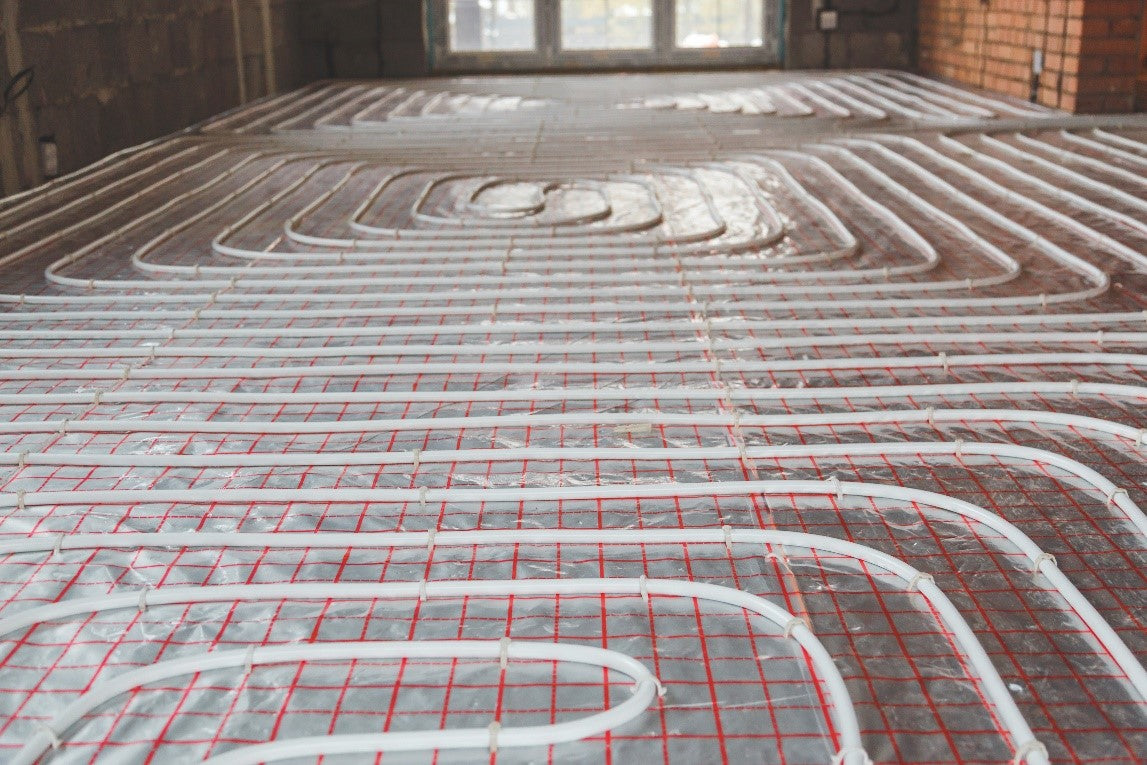We are a family run business that has specialised in underfloor heating systems & parts since 1981
View Basket | My Account | Checkout
Items: (0) | £0.00# How Do I Calculate How Much Pipe I Need For My Project Area?• You need to know what pipe centres you’re wanting your pipe to run at, by pipe centres we mean the distance or spacing between each pipe run you lay. So if you’re using a boiler, you would be running 200mm pipe centres. If you’re using a heat pump then you would be running 150mm pipe centres, sometimes 100mm centres depending on the project.
• You need to know how far the manifold is going to be away from each loop (room) plus 1 meter to go up the wall to connect to the manifold.
• Thirdly you need to calculate how much pipe you need for the area you require underfloor heating.

✅ 200mm centres you need to take the area and multiply it by 5.

Example:  Area (20m2) x 5 = 100m

✅ 150mm centres you need to take the area and multiply it by 6.6.

Example:  Area (30m2) x 6.6 = 198m

✅ 100mm centres you need to take the area and multiply it by 10.

Example:  Area (45m2) x 10 = 450m

Note: You need to do these calculations plus the distance from the manifold to the room (these are called the tails) plus that meter up the wall and multiply by 2 plus 5% for wastage

Example:  Area (20m2) x 5 = 100m

+ tails (5m) + meter up wall (1m) = 6m

x 2 = 12m

+ 5% = 12.6m

100m + 12.6m = 112.6m

So you would need a 150m pipe coil from us.

We hope this Underfloor Heating Pipe Calculator helped you calculate how much pipe you need for your area.

View our full range of Underfloor Heating Pipe.

If you have any questions or are still a little bit unsure, please do not hesitate to contact our technical help team on 01600 710909 or email technical@underfloorparts.co.uk and they will be more than happy to advise the correct amount of pipe needed.

0